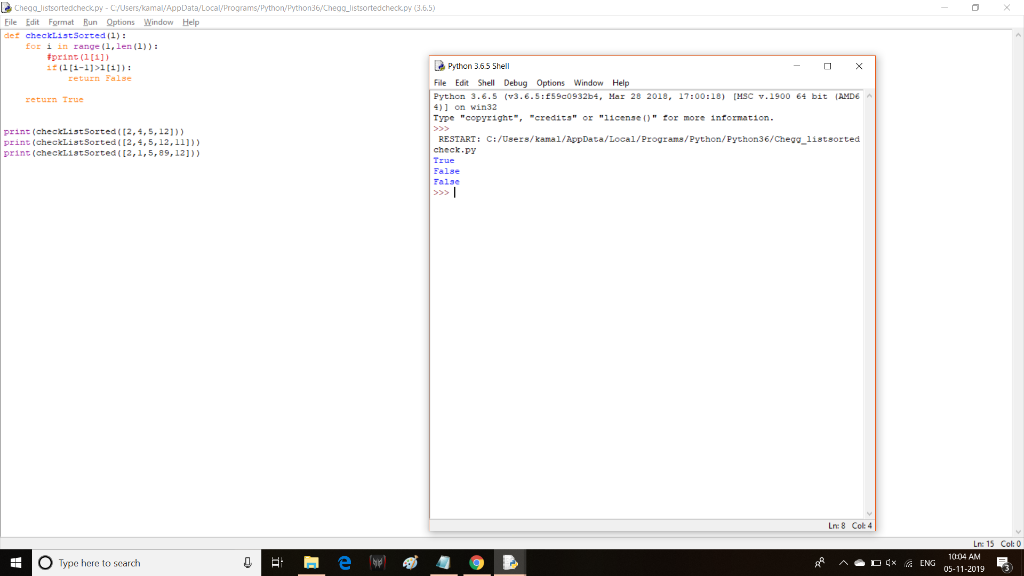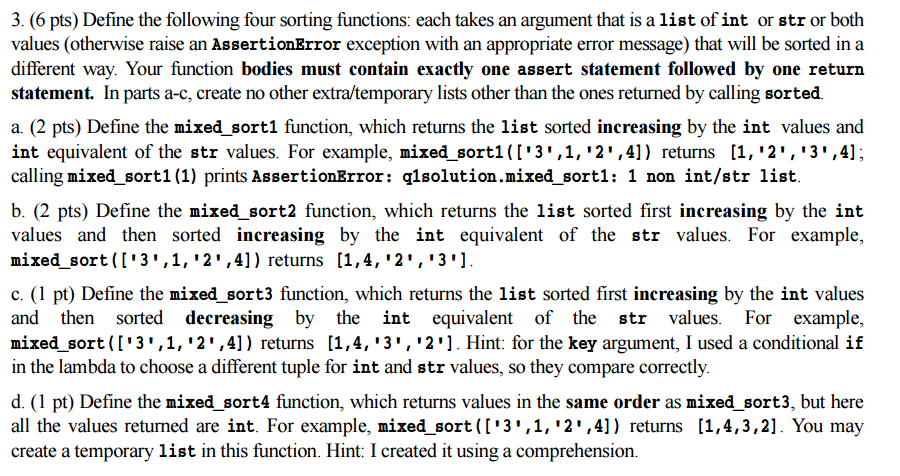Question
​Write a function that takes a list as an argument and returns true if the list is already sorted in increasing order. (You should not sort the list, and make sure that you just iterate over the list once! Only one loop!)
WRITE THE CODE IN PYTHON

python 3 code

============================================================================================

def checkListSorted(l):
for i in range(1,len(l)):
#print(l[i])
if(l[i-1]>l[i]):
return False

return True

print(checkListSorted([2,4,5,12]))
print(checkListSorted([2,4,5,12,11]))
print(checkListSorted([2,1,5,89,12]))

============================================================================================

Output#### Earn Coins

Coins can be redeemed for fabulous gifts.

Similar Homework Help Questions
• ### IN PYTHON: Write a function that takes, as an argument, a positive integer n, and returns...

IN PYTHON: Write a function that takes, as an argument, a positive integer n, and returns a LIST consisting of all of the digits of n (as integers) in the same order. Name this function intToList(n). For example, intToList(123) should return the list [1,2,3].

• ### Python 2.7 Write a function cumsum() that takes a list l as argument and returns the...

Python 2.7 Write a function cumsum() that takes a list l as argument and returns the cumulative sum (also known as the prefix sum) of l, which is a list, say cs of the same length as l such that each element cs[i] is equal to the sum of the first i + 1 elements of l, i.e., cs[i] == l + l + l + ... + l[i] You should not modify the argument list l in any way....

• ### Write a function count_vowels(s) that takes a string as an argument and returns the number of...

Write a function count_vowels(s) that takes a string as an argument and returns the number of vowels ('a', 'e', 'i' 'o', 'u') in the string. Should you use a for or while loop? (Implement this as a function, not as a class with a method.) Be sure to include unittest test cases to demonstrate that your code works properly, e.g Part 2: last_occurance(target, sequence) Write a function last_occurance(target, sequence) that takes two arguments: 1. target: A target item to find...

• ### Python problem. 3. (6 pts) Define the following four sorting functions: each takes an argument that...Python problem. 3. (6 pts) Define the following four sorting functions: each takes an argument that is a list of int or str or both values (otherwise raise an AssertionError exception with an appropriate error message) that will be sorted in a different way. Your function bodies must contain exactly one assert statement followed by one return statement. In parts a-c, create no other extra/temporary lists other than the ones returned by calling sorted. a. (2 pts) Define the mixed...

• ### Write a function findEvens that takes an integer, n, as input and returns the list of...

Write a function findEvens that takes an integer, n, as input and returns the list of even integers between 1 and n. Ex. Input: 10 Output: [2,4,6,8,10] Write a function sortList that takes in a list of strings and returns a list of those strings now sorted and lowercase. Ex. Input: [‘ABE’,’CAD’,’gaB’] Output: [‘abe’,’acd’,’abg’] Both done in Python

• ### Create a function that takes a list and an integer v, and returns True if v...

Create a function that takes a list and an integer v, and returns True if v is in the list (False otherwise). The function should be efficient and stop searching as soon as possible. •The main program must generate a very large list with random elements, call the function to search a value and display the result. Add in the function a variable Nsteps to count the number of steps used by the algorithm (number of times the loop is...

• ### in python Write a function that takes a string as an argument returns a new string...

in python Write a function that takes a string as an argument returns a new string that is that string repeated 3 times.

• ### Write this program in Scala: Write a function that returns true if a given list of...

Write this program in Scala: Write a function that returns true if a given list of integers is sorted in ascending order.

• ### write a Python function that takes in a list of integers and returns maximum and minimum values in the list as a tuple

1 write a Python function that takes in a list of integers and returns maximum and minimum values in the list as a tuple. Hint (can be done in one pass, you are not allowed to use built-on min and max functions.)max, min = find_max_min(my_list):2 write a Python function that takes in a list of integers (elements), and an integer number (num). The functions should count and return number of integers in elements greater than, less than, and equal to...

• ### Write a Python function isPrime(number) that determines if the integer argument number is prime or not....

Write a Python function isPrime(number) that determines if the integer argument number is prime or not. The function will return a boolean True or False. Next, write a function HowManyPrimes(P), that takes an integer P as argument and returns the number of prime numbers whose value is less than P. And then write a function HighestPrime(K) that takes integer K as an argument and returns the highest prime that is less than or equal to K. USE THE WHILE LOOP...